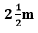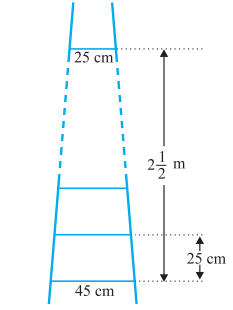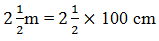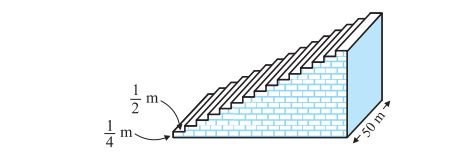Courses

# Exercise 5.4 NCERT Solutions: Arithmetic Progressions Notes | EduRev

## Class 10 : Exercise 5.4 NCERT Solutions: Arithmetic Progressions Notes | EduRev

The document Exercise 5.4 NCERT Solutions: Arithmetic Progressions Notes | EduRev is a part of the Class 10 Course Class 10 Mathematics by VP Classes.
All you need of Class 10 at this link: Class 10

Q.1. Which term of the AP: 121, 117, 113, . . ., is its first negative term? [Hint: Find n for an < 0]
Solution:
Given the AP series is 121, 117, 113, . . .,
Thus, first term, a = 121
Common difference, d = 117-121= -4
By the nth term formula,
an = a+(n −1)d
Therefore,
an = 121+(n−1)(-4)
= 121-4n+4
=125-4n
To find the first negative term of the series, a< 0
Therefore,
125-4n < 0
125 < 4n
n>125/4
n>31.25
Therefore, the first negative term of the series is 32nd term.

Q.2. The sum of the third and the seventh terms of an AP is 6 and their product is 8. Find the sum of first sixteen terms of the AP.
Solution:
From the given statements, we can write,
a3 + a7 = 6 …………………………….(i)
And
a×a= 8 ……………………………..(ii)
By the nth term formula,
an = a+(n−1)d
Third term, a= a+(3 -1)d
a= a + 2d………………………………(iii)
And Seventh term, a7= a+(7-1)d
a= a + 6d ………………………………..(iv)
From equation (iii) and (iv), putting in equation(i), we get,
a+2d +a+6d = 6
2a+8d = 6
a+4d=3
or
a = 3–4d …………………………………(v)
Again putting the eq.(iii) and (iv), in eq. (ii), we get,
(a+2d)×(a+6d) = 8
Putting the value of a from equation (v), we get,
(3–4d +2d)×(3–4d+6d) = 8
(3 –2d)×(3+2d) = 8
3– 2d2 = 8
9 – 4d2 = 8
4d2 = 1
d = 1/2 or -1/2
Now, by putting both the values of d, we get,
a = 3 – 4d = 3 – 4(1/2) = 3 – 2 = 1, when d = 1/2
a = 3 – 4d = 3 – 4(-1/2) = 3+2 = 5, when d = -1/2
We know, the sum of nth term of AP is;
Sn = n/2 [2a +(n – 1)d]
So, when a = 1 and d=1/2
Then, the sum of first 16 terms are;
S16 = 16/2 [2 +(16-1)1/2] = 8(2+15/2) = 76
And when a = 5 and d= -1/2
Then, the sum of first 16 terms are;
S16 = 16/2 [2.5+(16-1)(-1/2)] = 8(5/2)=20

Q.3. A ladder has rungs 25 cm apart. (see Fig. 5.7). The rungs decrease uniformly in length from 45 cm at the bottom to 25 cm at the top. If the top and the bottom rungs areapart, what is the length of the wood required for the rungs? [Hint: Number of rungs = -250/25 ].Solution:
Given,
Distance between the rungs of the ladder is 25cm.
Distance between the top rung and bottom rung of the ladder is == 5/2 ×100cm
= 250cm
Therefore, total number of rungs = 250/25 + 1 = 11
As we can see from the figure, the ladder has rungs in decreasing order from top to bottom. Thus, we can conclude now, that the rungs are decreasing in an order of AP.
And the length of the wood required for the rungs will be equal to the sum of the terms of AP series formed.
So,
First term, a = 45
Last term, l = 25
Number of terms, n = 11
Now, as we know, sum of nth terms is equal to,
Sn= n/2(a+ l)
Sn= 11/2(45+25) = 11/2(70) = 385 cm
Hence, the length of the wood required for the rungs is 385cm.

Q.4. The houses of a row are numbered consecutively from 1 to 49. Show that there is a value of x such that the sum of the numbers of the houses preceding the house numbered x is equal to the sum of the numbers of the houses following it. Find this value of x. [Hint :Sx – 1 = S49 – Sx ]
Solution:
Given,
Row houses are numbers from 1,2,3,4,5…….49.
Thus we can see the houses numbered in a row are in the form of AP.
So,
First term, a = 1
Common difference, d=1
Let us say the number of xth houses can be represented as;
Sum of nth term of AP = n/2[2a+(n-1)d]
Sum of number of houses beyond x house = Sx-1
= (x-1)/2[2.1+(x-1-1)1]
= (x-1)/2 [2+x-2]
= x(x-1)/2 ………………………………………(i)
By the given condition, we can write,
S49 – Sx = {49/2[2.1+(49-1)1]}–{x/2[2.1+(x-1)1]}
= 25(49) – x(x + 1)/2 ………………………………….(ii)
As per the given condition, eq.(i) and eq(ii) are equal to each other;
Therefore,
x(x-1)/2 = 25(49) – x(x-1)/2
x = ±35
As we know, the number of houses cannot be a negative number. Hence, the value of x is 35.

Q.5. A small terrace at a football ground comprises of 15 steps each of which is 50 m long and built of solid concrete. Each step has a rise of 1 4 m and a tread of 1 2 m. (see Fig. 5.8). Calculate the total volume of concrete required to build the terrace. [Hint : Volume of concrete required to build the first step = ¼ ×1/2 ×50 m3.]Solution:
As we can see from the given figure, the first step is ½ m wide, 2nd step is 1m wide and 3rd step is 3/2m wide. Thus we can understand that the width of step by ½ m each time when height is ¼ m. And also, given length of the steps is 50m all the time. So, the width of steps forms a series AP in such a way that;
½ , 1, 3/2, 2, ……..
Volume of steps = Volume of Cuboid
= Length × Breadth Height
Now,
Volume of concrete required to build the first step = ¼ ×1/2 ×50 = 25/4
Volume of concrete required to build the second step =¼ ×1/×50 = 25/2
Volume of concrete required to build the second step = ¼ ×3/2 ×50 = 75/2
Now, we can see the volumes of concrete required to build the steps, are in AP series;
25/4 , 25/2 , 75/2 …..
Thus, applying the AP series concept,
First term, a = 25/4
Common difference, d = 25/2 – 25/4 = 25/4
As we know, the sum of n terms is;
Sn = n/2[2a+(n-1)d] = 15/2(2×(25/4 )+(15/2 -1)25/4)
Upon solving, we get,
Sn = 15/2 (100)
Sn750
Hence, the total volume of concrete required to build the terrace is 750 m3.

Offer running on EduRev: Apply code STAYHOME200 to get INR 200 off on our premium plan EduRev Infinity!

132 docs

,

,

,

,

,

,

,

,

,

,

,

,

,

,

,

,

,

,

,

,

,

;